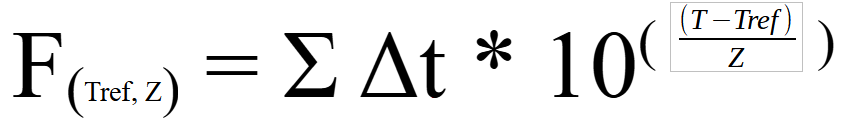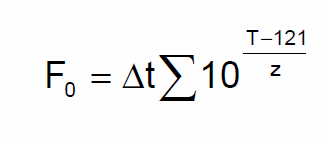# What formula do you use for F0?

The software that implement the lethality formula, TS Manager and SPD, use the international standard formula and both allow you to set the reference temperature (Tref), the Z value and the minimum temperature value to be considered for the contributions for the calculation. ending of lethality.

The implemented formula is the following:where is it:

t = time

T = temperature

In all our missions, of course, Δt is a constant.

The formula can therefore also be expressed in this way, by substituting the standard value for the reference temperature for the calculation of F0: